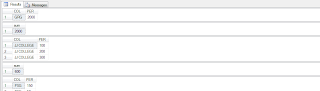# CUBE,COMPUTE,COMPUTE BY and ROLLUP OPERATOR IN SQL SERVER

The CUBE,COMPUTE,COMPUTE BY and ROLLUP operators are useful in generating reports that contain subtotals and totals.

There are extensions of the GROUP BY clause.

The result set of a ROLLUP operation has functionality similar to that returned by a COMPUTE BY; however, ROLLUP has these advantages:
ROLLUP returns a single result set; COMPUTE BY returns multiple result sets that increase the complexity of application code.

ROLLUP can be used in a server cursor; COMPUTE BY cannot.

The query optimizer can sometimes generate more efficient execution plans for ROLLUP than it can for COMPUTE BY.

### STEP 1:

create table JSSTUD(COL nvarchar(20),PER numeric(10))

### STEP 2:

INSERT INTO JSSTUD VALUES('JJ COLLEGE',100)
INSERT INTO JSSTUD VALUES('JJ COLLEGE',200)
INSERT INTO JSSTUD VALUES('JJ COLLEGE',300)
INSERT INTO JSSTUD VALUES('PSG',150)
INSERT INTO JSSTUD VALUES('PSG',50)
INSERT INTO JSSTUD VALUES('PSG',1050)
INSERT INTO JSSTUD VALUES('GRG',2000)

### STEP 3:

ROLLUP
SELECT COL,SUM(PER) FROM JSSTUD GROUP BY COL
WITH ROLLUPOR
COMPUTE bY

SELECT COL,PER FROM JSSTUD ORDER BY COL
COMPUTE SUM(PER) BY COLOR

SELECT COL,PER FROM JSSTUD ORDER BY COLCOMPUTE SUM(PER)